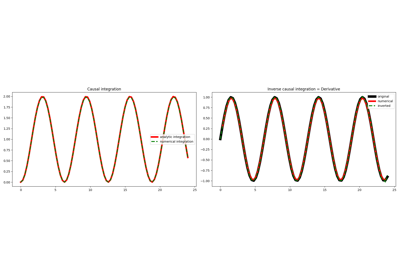# pylops.CausalIntegration#

class pylops.CausalIntegration(dims, axis=-1, sampling=1, kind='full', removefirst=False, dtype='float64', name='C')[source]#

Causal integration.

Apply causal integration to a multi-dimensional array along axis.

Parameters
dims

Number of samples for each dimension

axisint, optional

New in version 2.0.0.

Axis along which the model is integrated.

samplingfloat, optional

Sampling step dx.

kindstr, optional

Integration kind (full, half, or trapezoidal).

removefirstbool, optional

Remove first sample (True) or not (False).

dtypestr, optional

Type of elements in input array.

namestr, optional

New in version 2.0.0.

Name of operator (to be used by pylops.utils.describe.describe)

Notes

The CausalIntegration operator applies a causal integration to any chosen direction of a multi-dimensional array.

For simplicity, given a one dimensional array, the causal integration is:

$y(t) = \int\limits_{-\infty}^t x(\tau) \,\mathrm{d}\tau$

which can be discretised as :

$y[i] = \sum_{j=0}^i x[j] \,\Delta t$

or

$y[i] = \left(\sum_{j=0}^{i-1} x[j] + 0.5x[i]\right) \,\Delta t$

or

$y[i] = \left(\sum_{j=1}^{i-1} x[j] + 0.5x + 0.5x[i]\right) \,\Delta t$

where $$\Delta t$$ is the sampling interval, and assuming the signal is zero before sample $$j=0$$. In our implementation, the choice to add $$x[i]$$ or $$0.5x[i]$$ is made by selecting kind=full or kind=half, respectively. The choice to add $$0.5x[i]$$ and $$0.5x$$ instead of made by selecting the kind=trapezoidal.

Note that the causal integral of a signal will depend, up to a constant, on causal start of the signal. For example if $$x(\tau) = t^2$$ the resulting indefinite integration is:

$y(t) = \int \tau^2 \,\mathrm{d}\tau = \frac{t^3}{3} + C$

However, if we apply a first derivative to $$y$$ always obtain:

$x(t) = \frac{\mathrm{d}y}{\mathrm{d}t} = t^2$

no matter the choice of $$C$$.

Attributes
shapetuple

Operator shape

explicitbool

Operator contains a matrix that can be solved explicitly (True) or not (False)

Methods

 __init__(dims[, axis, sampling, kind, ...]) adjoint() apply_columns(cols) Apply subset of columns of operator cond([uselobpcg]) Condition number of linear operator. conj() Complex conjugate operator div(y[, niter, densesolver]) Solve the linear problem $$\mathbf{y}=\mathbf{A}\mathbf{x}$$. dot(x) Matrix-matrix or matrix-vector multiplication. eigs([neigs, symmetric, niter, uselobpcg]) Most significant eigenvalues of linear operator. matmat(X) Matrix-matrix multiplication. matvec(x) Matrix-vector multiplication. reset_count() Reset counters rmatmat(X) Matrix-matrix multiplication. rmatvec(x) Adjoint matrix-vector multiplication. todense([backend]) Return dense matrix. toimag([forw, adj]) Imag operator toreal([forw, adj]) Real operator tosparse() Return sparse matrix. trace([neval, method, backend]) Trace of linear operator. transpose()

## Examples using pylops.CausalIntegration#Causal Integration

Causal Integration